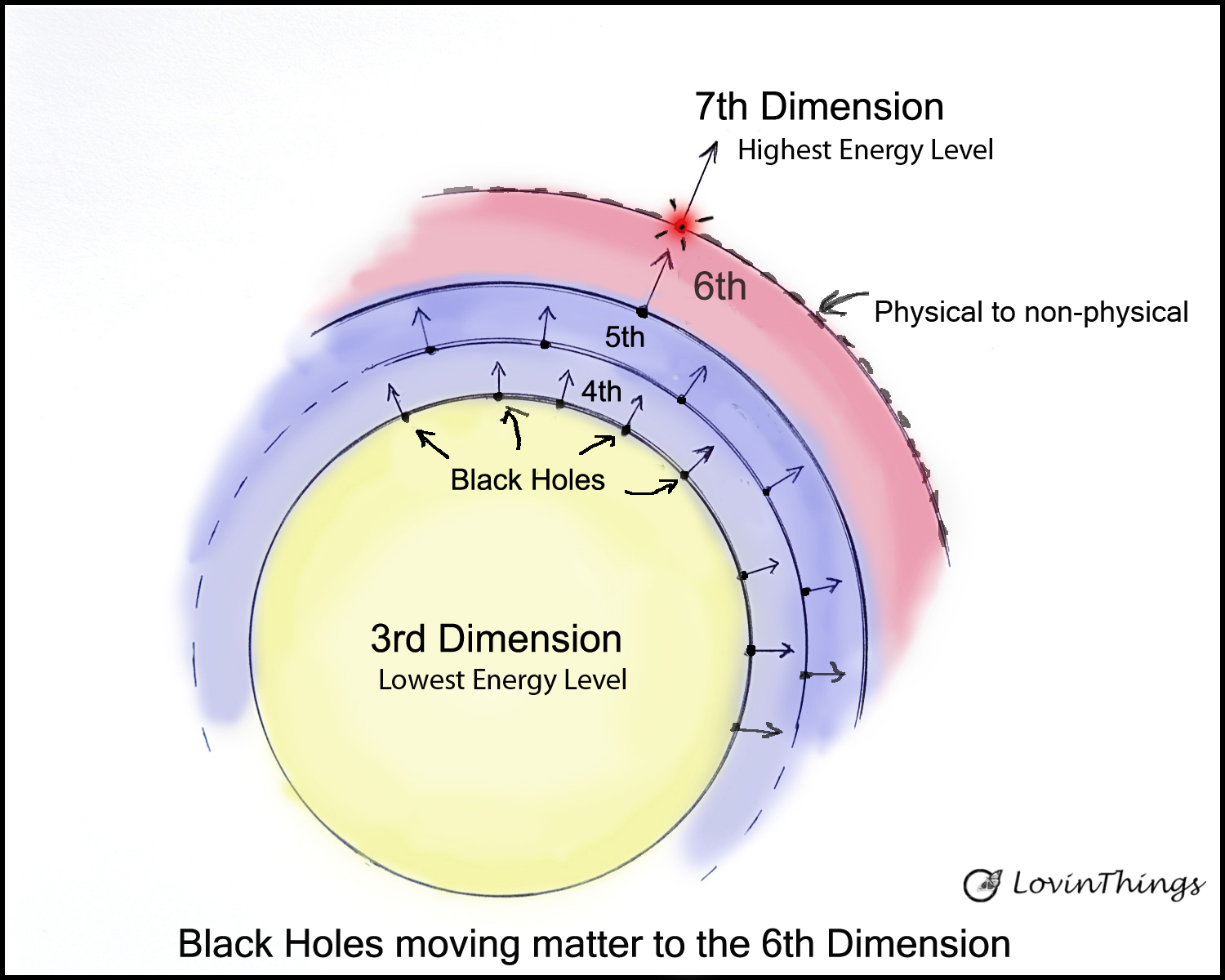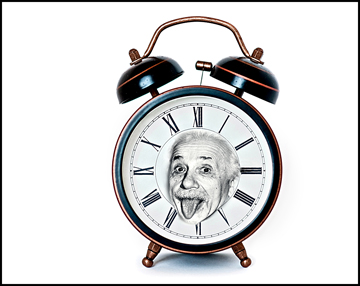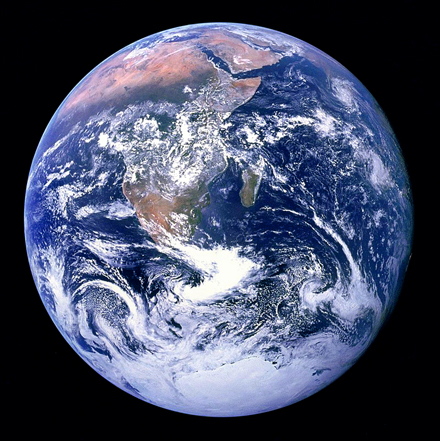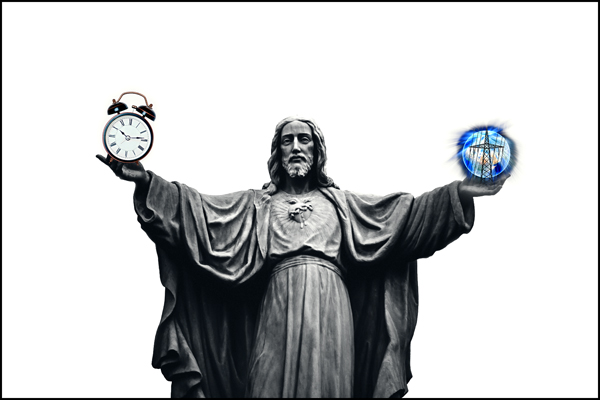# Believe This, Time is Moving Faster in 2021

## Believe This, Time is Moving Faster in 2021

Time has been moving forward at a constant speed as long as we have kept records but I think time has been slowly moving faster since the late 1980s. I haven’t felt time moving faster until this year. In this article, I will examine if time is moving faster in 2021 and beyond.We tend to think that everything in the universe exists in the observable 3rd dimension. But the universe is much larger than the low energy-level of our 3rd dimension. Can time move faster, and how is that possible?

## Measuring the speed of Motion

Nikola Tesla said, “ To understand the universe, think in terms of energy, frequency, and vibrations”.Light travels about 3 x10^8 m/sec in the 3rd dimension but is this speed invariant?

The speed of light must be the same in a higher energy level (the 4th dimension) if it’s truly invariant and non-changing.

But can light move faster in other energy levels (dimensions) of space or does it just have a higher frequency?

It takes the Cesium-133 atom in an atomic clock one second to oscillate 9,192,631,770 vibrations (motion), and one second is equal to 1/86,400th of a mean Earth day.

Suppose a mind experiment that in a higher energy level (dimension) the Cesium atom vibrates two times faster (double the frequency).

That means what took one second in the 3rd dimension for the vibrations of a Cesium-133 atom now takes half as long.

Do you think the measure of a clock’s time and the speed of light move two times faster?

Is the speed of light related to the speed of a clock’s time or is the speed of motion from some other source?

If our definition of motion is based on the frequency of the Cesium atom then we won’t be able to tell if motion is moving faster.

Each moment the Cesium-133 atom counts the correct amount we believe it’s one second.## The Error of Time

Does a faster frequency mean that the motion of light is moving twice as fast?

If we measure the speed of light we might find that light has twice the frequency of the old light but it’s moving at half the wavelength, leaving the speed of light “c” unchanged.

It seems that time changes at the same rate as motion changes. In a faster frequency of energy, does the motion of light move two times farther than before? I don’t think so.

If an electron emits a photon that travels at the speed of “c” and then if the same electron has a frequency that’s two times faster does the speed of a photon change?

I think that the wavelength becomes half the length and the frequency becomes two times greater so that the speed of light remains the same?

But like I said we have no way of knowing if the frequency of energy is moving faster, except we feel that time is going by faster.

What happens when we measure the motion of light using the same Cesium-133 frequency as before?

When the Cesium clock measures 9,192,631,770 vibrations (in half the old rate) then we have measured that light moves at the same speed as before.

We wouldn’t know that the Cesium-133 atom is oscillating two times faster than the old speed because we are using its frequency of motion as our standard.

After 9,192,631,770 vibrations we will measure that one second has been counted because that’s how we measure time/motion.

If we use the same number of vibrations of the cesium-133 atom we will always measure that the speed of light is constant even if it’s moving faster.

## Does the Speed of Light Change?

Does the speed of light change or is it only an error in the measurement of a second?

I think, the light just has two times the energy with two times the frequency and half the wavelength to always give the same value for “c”?

It doesn’t make sense to talk about time having energy or force to change anything.

Time is a number and has no energy to move things faster or slower. It’s not time that’s changing, it's only the motion that’s changing and time is measured by that motion.

In half of a second does the Earth rotate the same amount as it did before in one second?

How can we measure motion without using time or frequencies? We have invented the time of one second to mean 9,192,631,770 oscillations of an atom.

I don’t know how to get around this paradox? Could we measure the wavelength or frequency of new light and compare it with older data of the same kind of light?

## The Earth is Moving FasterIf motion moves two times faster does the Earth spin two times faster as well?

I think that the Earth would rotate two times faster if the motion was moving two times faster but time would be the same.

The clocks would tick faster so that each day is still 86,400 seconds.

We would not know the days are getting shorter because we see the same sunrise and sunset as before except it feels like the days move faster.

Don’t you think that the days are getting shorter? Maybe the second is shorter by a small amount already so the Earth spins a few minutes faster and that’s why the weeks seem to pass so fast?

How can time be faster since the Earth rotates in one day and moves around the sun in one year, right?

Well, the Earth can spin around its axis twice as fast and if the Earth moves faster and closer to the sun for its rotation then our measurements make it look like everything is normal.

Every measurement of motion and distance will be the same because we have standardized time with the frequency of atoms.

However, we would notice climate changes and feel like the days are getting shorter. Also, we would notice that we live longer but that's another paradox.

How can we find out the truth? How can we tell if motion and “time” are moving faster?

We use the same frequency of Cesium-133 to measure motion and if that changes we still measure time and the speed of light as before. How can we solve this paradox?

What is real-time or does it matter?

If we use a pendulum clock adjusted to the new rate of one second we wouldn’t know time is moving faster.

But if we don’t adjust a pendulum clock’s motion and later compare it to the atomic clock’s time will we see a difference?

A pendulum clock only relies on the force of gravity and not on frequencies.

If the frequency of matter is faster, then our measurement of the motion is false.

We will sense that the days seem shorter but everything else looks normal so we assume the reason is because of our old age?

Yes, Einstein, time is relative. This shows how malleable time is. What does the speed of motion say about our biological age?

If energy is moving faster we will seem to live more years but these are shorter years that aren’t equal to our “real” biological age.

The health statistics report that our average age is getting longer, but is it?

## Proof That Motion is Moving Faster

Millimetre-precision measurements of the lunar distance are made by measuring the time it takes for light to travel between LIDAR stations on the Earth and retroreflectors placed on the Moon.

The Moon is spiraling away from the Earth at an average rate of 3.8 cm (1.5 in) per year, as detected by the Lunar Laser Ranging Experiment.

What does this mean? Is the moon moving away from Earth slowly or is the frequency of light moving faster?

Here is my take on that info. Suppose motion and frequencies are moving at a faster speed.  What would that do to the measurement of the moon’s distance from Earth?

Normally it takes about 2 and 1/2 seconds for light to travel to the moon and back based on the speed of “c” and our standard time of a second.

Note: we are using time to measure the speed and the distance but what if the frequency of motion is now moving 2 times faster?

Light and other “massless” objects do not move faster because the speed of light is fixed at E=mc2.

So to double the energy of the new light it will have 2 times greater frequency.

During the new speed of “time” light will travel half as far in half the “time” which means the speed “c” remains the same but at twice the frequency.

Speed “c” = distance divided by time. New speed of “c” = 0.5 distance divided by 0.5 time. The speed of light remains the same but at a higher frequency.

Therefore, the distance to the moon remains the same and the greater distance of 1.5 inches per year could be caused by some other force.

## Believe This, Time is ChangingThe measurement on a clock will change if a clock is in a different field of gravity, this is called time dilation and is a proven fact.

But time dilation doesn’t change motion because time is a number on a clock and has no power to do anything.

If the frequency of matter is greater then the motion of energy will change and a clock will change with that frequency.

Time is moving faster as our solar system moves into higher frequency areas of the universe.

I want everyone to put on their thinking caps (minds) and find ways to prove that time is moving faster because of frequency changes.

Thanks for your time here, visit me at https://lovinthings.com/  If you have any ideas please send them to eriklovin@gmail.com

Be well and be safe oxo

### About the Author Erik Lovin

Erik has a BSc degree and is a retired professional photographer who is now a published Author of many books. His passion is understanding how life and the universe works. He is currently blogging about the science of the Big Bang and the science of cosmology. Erik is helping his tribe with questions about the universe. His goal is to help find a theory of everything (TOE). In order to do that, he is trying to prove light has mass and that the fabric of spacetime is a false theory. We are welcoming questions and answers that you might have about the universe.